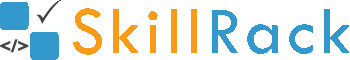Solution Required #include using namespace std; int main() { // your code goes here int a=5; cout<<(++a + ++a + ++a) ; return 0; }# #include using namespace std; int main() { // your code goes here int a=5; cout<<(++a + ++a + ++a) ; return 0; }

Solution RequiredAbinesh
0 5 258
3 years ago

#include <iostream>
using namespace std;

int main() {
int a=5;
cout<<(++a + ++a + ++a) ;
return 0;
}


aravind G
0 4 361
3 years ago

22 is the answer when the evaluation is from right to left so values will be 8 + 7 +6 when 6+7 is added it turns as 7+7 and 14 is returned when 14 is added with 8 22 is printed
NARESH
21 12 2842
3 years ago

All this confusion arises because of operator + + is more like a function which accepts arguments i.e: a+b --> +(a,b) so ++a + ++a --> +(++a,++a) //both are same after compilation: ++a + ++a + ++a =22 ++a +(++a + ++a)=24 (++a + ++a)+ ++a=22 This is all because the function accepts final value only: x=5 add(++x,++x); //it should pass as add(6,7) but it passes as: add(7,7) //becauz only after calculation of x is the value passed. thus ++a + ++a + ++a (7+7)+ ++a 14 + 8 = 22
NARESH
21 12 2842
3 years ago

Last reply is the answer Here are examples to better understand: Not in the same comment since there is a limit of 500 characters cout and calculation are performed in left to right order and fuction arguments from right to left order (above are found from experimentation of online compiler) More example reason for experimental conclusion are attached with the picture.
R ANUSHA
0 0 125
3 years ago

Here the operation begins from right towards left. so at first ++a= 6; then ++a + ++a=7+6 but instead it takes the calculated value of' a' so it becomes 7+7 and then now value of 'a' is 7. then (1+7) +14=8+14. thus the output would be 22
VISHAL
3 12 2507
3 years ago

21
DHIVYA
0 0 381
3 years ago

27
Hemakaviya. S
0 0 112
3 years ago

a=5 (++a + ++a) + ++a//(6,7) (7,7)+ ++a 14+8= 22 operators right to left
PRAVEEN KUMAR. S
0 1 319
3 years ago

this is the ans
KALAIVANI G
0 0 84
3 years ago

24
BAVIREDDY VENKATA MANISH BABU
0 0 113
2 years ago

22